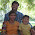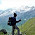## Power Planning - Power Network Synthesis (PNS)

Power Planning - Power Network Synthesis (PNS)

In ICC Design Planning flow, Power Network Synthesis creates macro power rings, creates the power grid. PNS automates power topology definition, Calculations of the width and number of power straps to meet IR constraints, detailed P/G connections and via placement.

Here I am going to discuss about the Calculations of the width and number of power straps to meet EM IR constraints.Suppose consider core voltage Vdd core = 1.2volts.

Using below mentioned equations we can calculate vertical and horizontal strap width and required number of power straps.

1. Calculation of block currents w.r.t to power:

Iblock = Pblock/ Vddcore

Where Pblock = Block Power
Vdd core = Core Voltage

2. Calculation the current supply from each side of the block :

Itop= Ibottom= {Iblockx [Wblock / (Wblock+Hblock)]} / 2

Ileft= Iright= {Iblock x [Hblock/ (Wblock+Hblock)]} / 2

3. Calculation of power-strap width based on EM:

W strap_vertical( = W strap_top= W strap_bottom) = Itop/ J metal

W strap_horizontal( = W strap_left= W strap_right) = Ileft/ J metal

4. Calculation of strap width based on IR drop dominates:

Wstrap_vertical ≧ (Itop x Roe x Hblock) / 0.1Vdd

Wstrap_horizontal≧ (Ileftx Roe x Wblock) / 0.1Vdd

5. Partition the power straps into power refreshes:

For better utilization of the routing channels, select a refresh width of (3 routing pitch + minimum metal6 width) = (3 x 0.59 μm + 0.25 μm) = 2.01μm 2 μm in the vertical and the same in the horizontal.

Block A as an example, the number of the Vdd/Vssrefresh is:

Nrefresh_horizontal= Wstrap_ horizontal/ Wrefresh

Nrefresh_vertical= Wstrap_vertical / Wrefresh

The spacing of each refresh would be:

Srefresh_horizontal= Hblock/ Nrefresh_horizontal

Srefresh_vertical = Wblock/ Nrefresh_vertical

6. Calculate the required number of core power/ground pads:

If each power/ground pad can sustain 25 mA current, Pcore=630mw

= (630/1.2)/25
= 21

7. Core Power Estimation :

The following equation provides a simple method to estimate the dynamic power and leakage power of combinational cells in the core area:

Pdynamic= Pcore x F x Scomb x Ncomb

Where,
Pcomb. is the power per MHz per gate count (nW/MHz/gate)
F is the working frequency. (Unit = MHz)
Scomb. is the switching activity of combinational logic

Ncomb. is the number of gate counts

Pstatic= Pleakagex Ncomb

Where,
Pleakage is average leakage power of gate
Ncomb. is the number of gate counts
Consider
•Gate count of combinational logic is 160K gates
•The working frequency is 27MHz
•Switching activity is 0.2

Then, the dynamic power consumption in the combinational circuit is,
Pdynamic = Pcorex F x Scombx NcombPdynamic
= 12.35 nW/MHz X 27 X 0.2 * 160K
= 10.67 mW

The leakage power consumption in the combinational logic is
Pstatic= Pleakagex NcombPstatic
= 0.756 nW X 160K
= 0.121 mW

1.2.